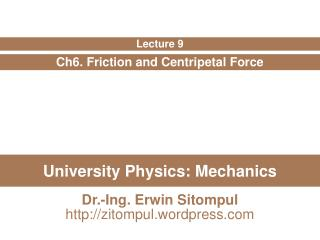DownloadDownload PresentationUniversity Physics: Mechanics

University Physics: Mechanics

Download PresentationUniversity Physics: Mechanics

- - - - - - - - - - - - - - - - - - - - - - - - - - - E N D - - - - - - - - - - - - - - - - - - - - - - - - - - -
Presentation Transcript

1. University Physics: Mechanics Ch6. Friction and Centripetal Force Lecture 9 Dr.-Ing. Erwin Sitompul http://zitompul.wordpress.com

2. Homework 7: Coin On A Book The figure below shows a coin of mass m at rest on a book that has been tilted at an angle θ with the horizontal. By experimenting, you find that when θ is increased to 13°, the coin is on the verge of sliding down the book, which means that even a slight increase beyond 13° produces sliding. What is the coefficient of static friction μs between the coin and the book? Hint: Draw the free-body diagram of the coin first.

3. Solution of Homework 7: Coin On A Book Forces along the y axis: • Why zero? Forces along the x axis: • Why zero? So, the coefficient of static friction is:

4. Example: Blue Block → A block of mass m = 3 kg slides along a floor while a force F of magnitude 12 N is applied to it at an upward angle θ. The coefficient of kinetic friction between the block and the floor is μk = 0.4. We can vary θ from 0 to 90° (with the block remains on the floor. What θ gives the maximum value of the block’s acceleration magnitude a?

5. Example: Blue Block Forces along the y axis: Forces along the x axis: • What θ gives the maximum value of a? • da/dθ = 0

6. Example: Blue Block If a is given by then, the derivative of a with respect to θ is

7. Example: Two Blocks Block B in the figure below weighs 711 N. The coefficient of static friction between block and table is 0.25; angle θ is 30°. Assume that the cord between B and the knot is horizontal. Find the maximum weight of block A for which the system will be stationary.

8. Example: Two Blocks → → → → → → → → → → → → → fs,max fs,max TW FgB FNB FgA TB TW FgA TA TW TB TA Wall Knot Block A Block B Knot

9. Example: Two Blocks → → → fs,max TW FgA Forces along the y axis: TWy θ TWx Knot Forces along the x axis:

10. Example: Multiple Objects A block of mass m1 on a rough, horizontal surface is connected to a ball of mass m2 by a lightweight cord over a lightweight, frictionless pulley as shown in the figure below. A force of magnitude F at an angle θwith the horizontal is applied to the block as shown and the block slides to the right. The coefficient of kinetic friction between the block and surface is μk. Find the magnitude of acceleration of the two objects.

11. Example: Multiple Objects → → → → → → → fk FN Fg2 F T T Fg1 Fy θ Fx m1 Forces in m1 Forces in m2 m2

12. Example: Multiple Objects

13. Example: Trio Blocks When the three blocks in the figure below are released from rest, they accelerate with a magnitude of 0.5 m/s2. Block 1 has mass M, block 2 has 2M, and block 3 has 2M. What is the coefficient of kinetic friction between block 2 and the table?

14. → → → → → → → → fk T1 T1 Fg1 Fg2 FN T2 T2 Fg3 Example: Trio Blocks a Forces in m1 Forces in m2 a a Forces in m3 m1 m2 m3

15. Example: Trio Blocks

16. Homework 9 • In the next figure, blocks A and B have weights of 44 N and 22 N, respectively. • Determine the minimum weight of block C to keep A from sliding if μs, between A and the table is 0.20. • Block C suddenly is lifted off A. What is the acceleration of block A if μk between A and the table is 0.15? New